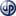When you were in math class during elementary/primary school, you probably weren’t thinking about how the lessons on addition and subtraction could be applied to life. But yes, life lessons can be found in basic arithmetic. Because life is like a number line accompanied by one long equation of plus and minus signs.

So, welcome to Life Lessons in Math 101.

Before we begin, there are mathematical principles and connections we want to establish.

1. First, there exists a number line that every number fits into. To the left of zero on the number line are negative numbers, and to the right are positive numbers. We will be imagining life as a number line.
2. Second, in front of every number greater than zero is an invisible plus sign indicating that it’s positive. Meanwhile, in front of every number below zero is a minus sign indicating that it’s negative. Positive numbers will symbolize the positives in life and negative numbers will symbolize the negatives.

From this framework, we can find the following life lessons.

#### Life Lessons in Basic MathCopyright secured by Digiprove © 2023